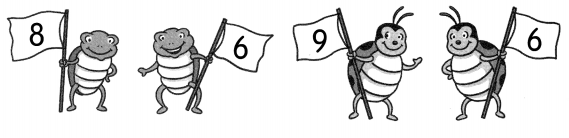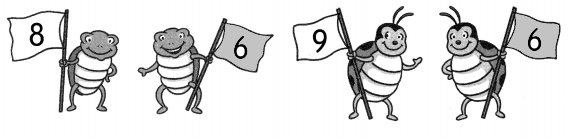# Math in Focus Grade 1 Chapter 1 Practice 2 Answer Key Comparing Numbers

This handy Math in Focus Grade 1 Workbook Answer Key Chapter 1 Practice 2 Comparing Numbers detailed solutions for the textbook questions.

## Math in Focus Grade 1 Chapter 1 Practice 2 Answer Key Comparing Numbers

Count.

Circle the groups that have the same number.

Example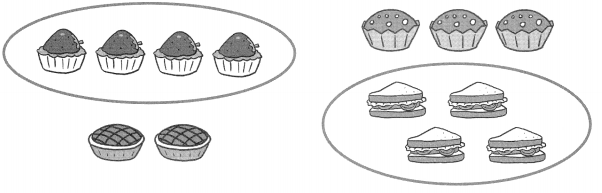Question 2.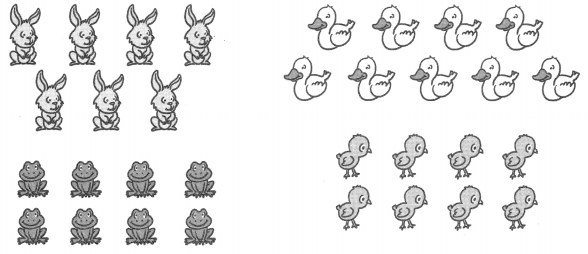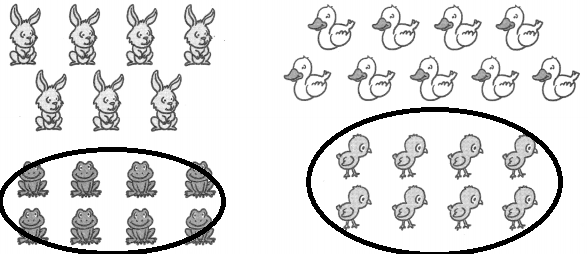Explanation:
Number of frogs = number of ducks
8 = 8
In the first group there are 7 bunnies and in the second group there are 9 ducks
and in the remaining two group there are 8 frogs and 8 ducks.

Match. Then circle the answer to each question.

Example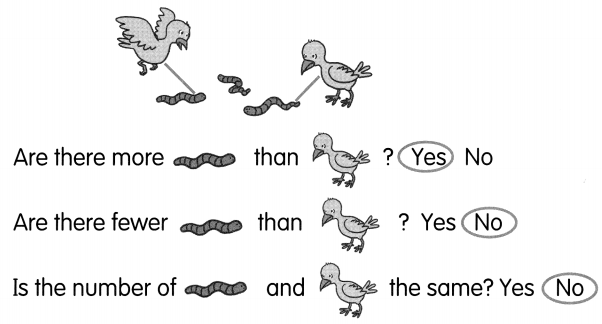Question 3.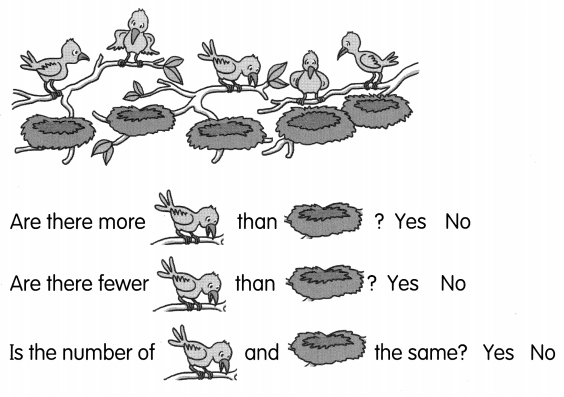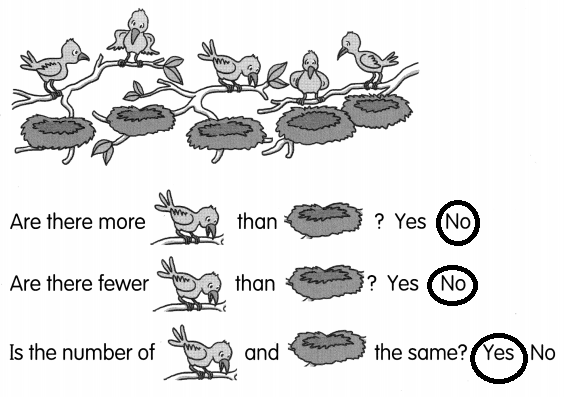Explanation:
Total number of birds are 6 and the nests are also 6
6 = 6

Question 4.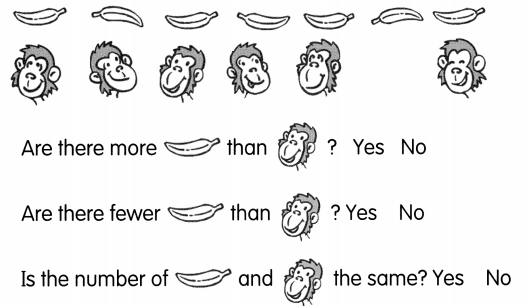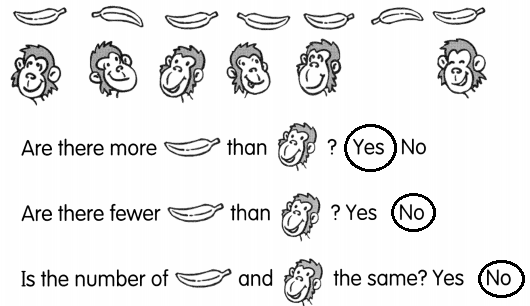Explanation:
The number of monkeys and the bananas are not equal
7 6
there are 7 bananas and 6 monkeys.

Question 5.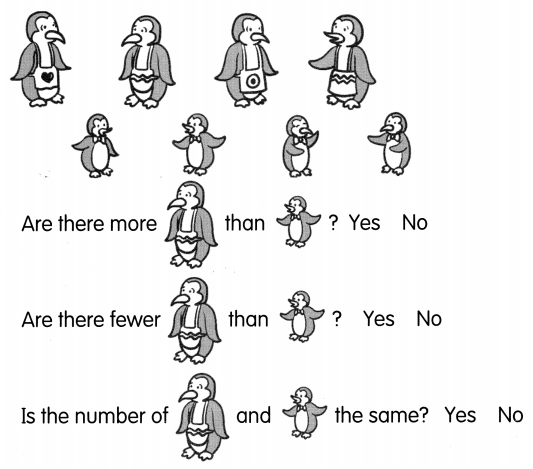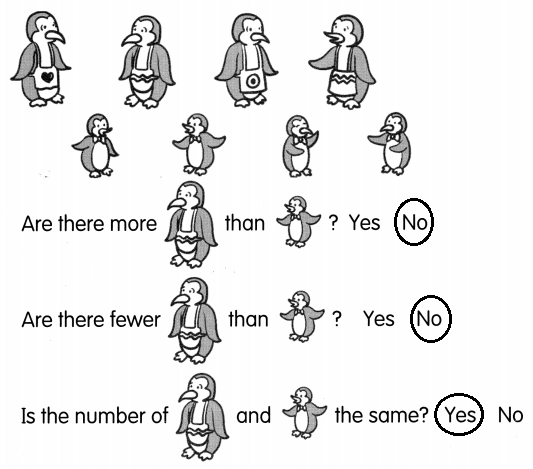Explanation:
The penguins and there babies are in same number.
mothers = babies
4 = 4

Which two groups have the same number of things?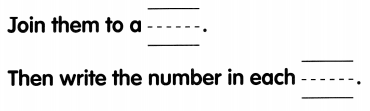Question 6.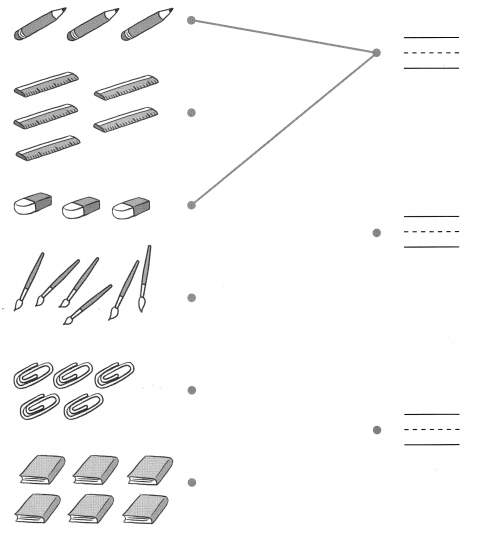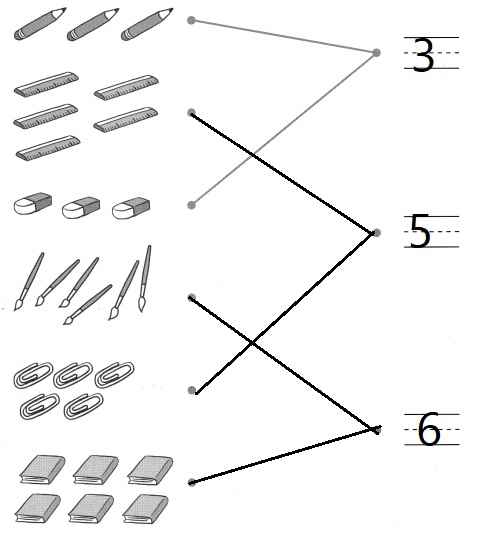Explanation:
The things with the same quantity are matched and marked with the numbers.

Count and write the number. Then answer each question by coloring the correct box.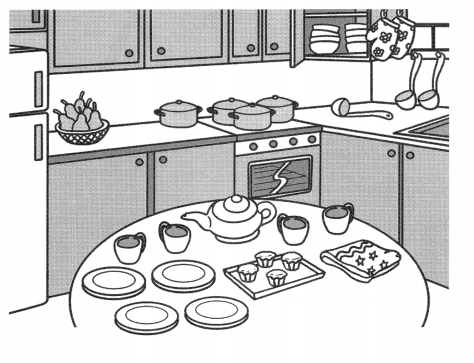Which is more?

Example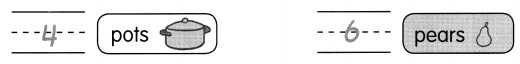Question 7.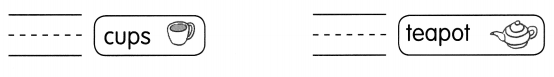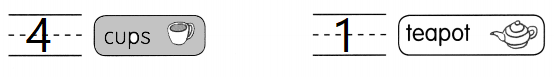Explanation:
In the above picture there are 4 cups and 1 tea pot
as 4 is greater than 1

Which is fewer?

Question 8.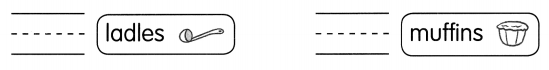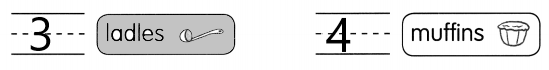Explanation:
In the above picture there are 3 ladles and  4 muffins
as 3 is lesser than 1

Question 9.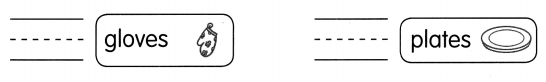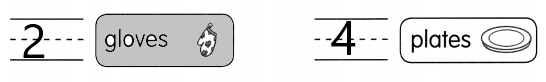Explanation:
In the above picture there are 2 gloves and  4 plates
2 is lesser than 4

Color the correct signs.

Which number is greater?

Question 10.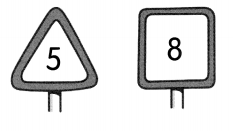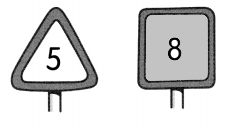Explanation:
The triangle sign is showing number 5 and the square is showing number 8
shaded the number 8 as it is greater than 5

Question 11.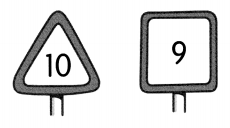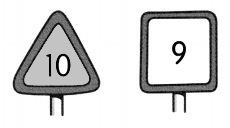Explanation:
The triangle sign is showing number 10 and the square is showing number 9
shaded the number 10 as it is greater than 9

Which number is less?

Question 12.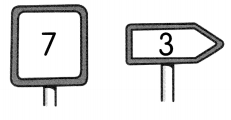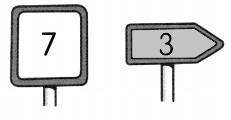Explanation:
The square sign is showing number 7 and the arrow is showing number 3
shaded the number 3 as it is lesser than 7

Question 13.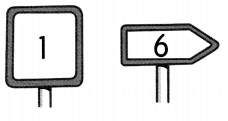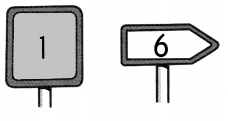Explanation:
The square sign is showing number 1 and the arrow is showing number 6
shaded the number 1 as it is lesser than 6

Write the numbers in the blanks.

Question 14.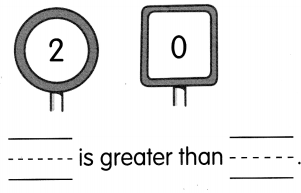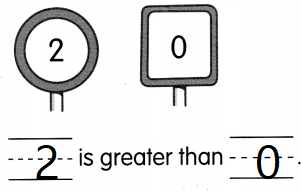Explanation:
In the circle it is 2 and in the square it is 0
2 > 0
2 is greater than 0

Question 15.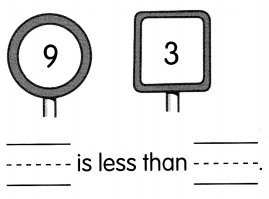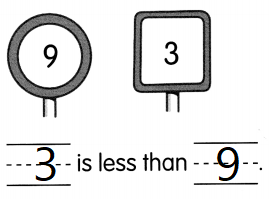Explanation:
In the circle it is 9 and in the square it is 3
3 < 9
3 is lesser than 9

Color the flags with the same number.

Question 16.